## 1-Calc evaluates the simplest and most complex math expressions both as fractions and in decimal format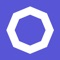# 1-Calc : Graphing Financial and Scientific Calculator with Numerical Solver and Optimizer

by Petri Jokinen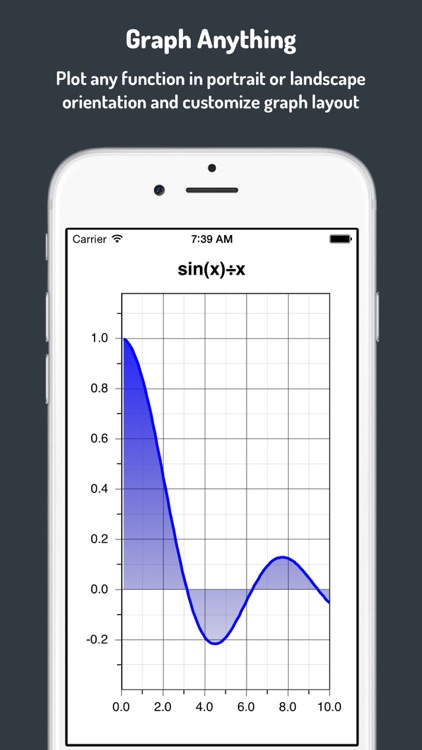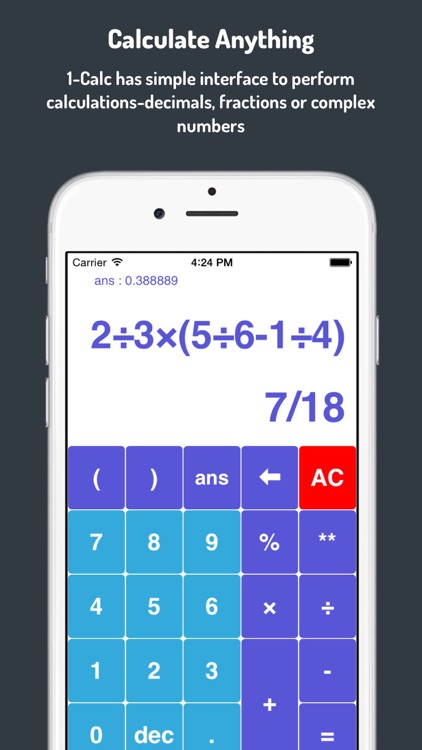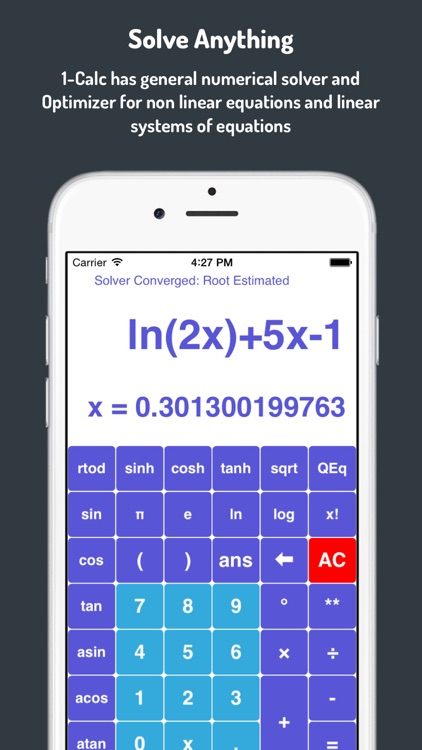1-Calc evaluates the simplest and most complex math expressions both as fractions and in decimal format.Version
1.9.5
Rating
NA
Size
7Mb
Genre
Last updated
January 28, 2016
Release date
May 5, 2015

### App Screenshots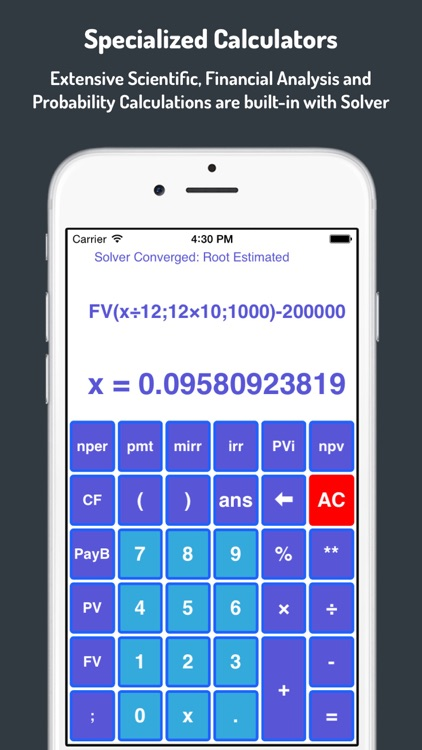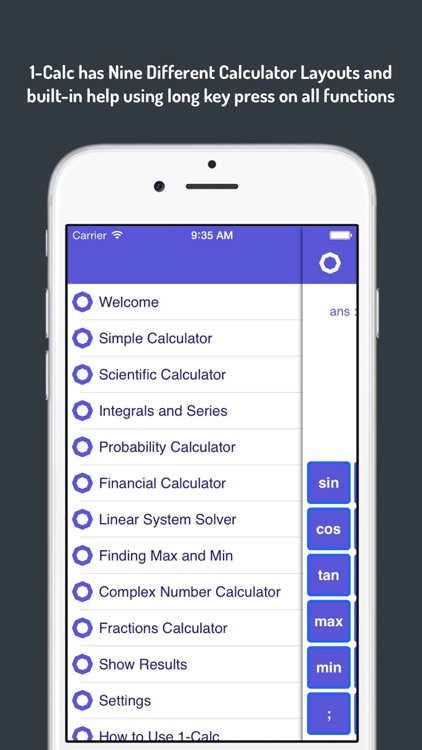### App Store Description

1-Calc evaluates the simplest and most complex math expressions both as fractions and in decimal format.

Flexible graphing of any function in either portrait or landscape orientation. Numerical integration and differentiation of functions, calculation of limit values and partial sums of series can be performed on any real valued function.

Built-in numerical solver and optimiser extend the 1-Calc functionality beyond other calculators. Calculations related to probability, financials, linear systems of equations and even complex number arithmetic are all there for you.

Long Press on any calculator key opens a help screen giving background information on usage of the operation or function and detailed instructions. 1-Calc Calculator layouts can be varied selecting display attributes, a total of 512 different layouts are available.

1-Calc has currently Ten Specialised Calculators that can be used depending on the nature of problems you are solving most often:

• Simple Calculator has the common arithmetic operations: addition, subtraction, multiplication, division and exponentiation. Expressions can be edited with delete key and calculation order defined using unlimited parenthesis levels. The unique feature of even this simplest calculator is the ability to numerically solve expressions containing single variable x.
• Fractions Calculator has the same arithmetic calculation functionality as the Simple Calculator. All calculations are performed using rational number representation as fractions. The fractional results can also be converted to decimal representation.
• Graphing Calculator has all the functionality of the Simple Calculator and adds the commonly used logarithmic, exponential, hyperbolic, trigonometric and inverse trigonometric functions with graphing of any function. A quadratic equation solver can solve all real and imaginary roots of quadratic equation with real coefficients.
• Integrals and Derivates Calculator has majority of the same special functions as the Scientific Calculator and adds numerical integration and derivation of user definable functions. The functions can be integrated on definite intervals and also intervals either starting from negative infinity or stopping to infinity (or both).
• Limits and Sums Calculator has the capability to calculate limit values of user definable functions. The function limit values can be evaluated to negative or positive infinity or any other point.
• Probability Calculator contains all the common probability distribution functions such as Normal, Weibull, Geometric, Binomial, Negative Binomial and Hypergeometric distributions. Calculation functions for permutations and combinations are also included.
• Financial Calculator can perform all annuity, mortgage and profitability calculations. Cash flows can be defined for performing net present value (NPV), profitability index (PV/I), payback period, internal rate of return (IRR) and modified internal rate of return (MIRR) calculations.
• Finding Maximum and Minimum can be performed for any non-linear function by using Optimization Calculator. 1-Calc solves both constrained and unconstrained optimisation problems using Nelder-Mead method.
• Linear System Solver can solve underdetermined, square and overdetermined linear systems of equations. 1-Calc solves these problems as linear least squares problem while minimising 2-norm. Singular Value Decomposition (SVD) method is used. The solver is able to calculate systems of equations with up to twenty variables and 50 equations.
• Complex Number Calculator has all arithmetic calculation functionality for complex numbers including exponential, logarithmic and trigonometric functions.
• Moving Between Calculators is easy. Just swipe left or right to move to the previous or next calculator and swipe down to expose the navigation bar.
• Export Results as e-mail attachment or by sending them out using Twitter directly from 1-Calc.

Disclaimer:
AppAdvice does not own this application and only provides images and links contained in the iTunes Search API, to help our users find the best apps to download. If you are the developer of this app and would like your information removed, please send a request to [email protected] and your information will be removed.